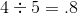SSAT Elementary Level Math : How to find the decimal equivalent of a fraction

Example Questions

Example Question #41 : How To Find The Decimal Equivalent Of A Fraction

What is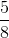equal to in decimal form?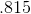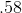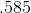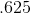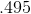Explanation:

To find the decimal equivalent of a fraction, divide the top number (numerator) by the bottom number (denominator). In this problem,divided byequals.

Example Question #42 : How To Find The Decimal Equivalent Of A Fraction

What isequal to in decimal form?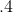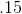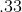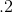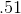Explanation:

To find the decimal equivalent of a fraction, divide the top number (numerator) by the bottom number (denominator). In this problem,divided byequals.

Example Question #43 : How To Find The Decimal Equivalent Of A Fraction

What is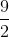equal to in decimal form?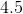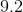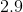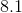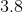Explanation:

To find the decimal equivalent of a fraction, divide the top number (numerator) by the bottom number (denominator). In this problem,divided byequals.

Example Question #44 : How To Find The Decimal Equivalent Of A Fraction

Convert the fraction to a decimal, rounded to two places: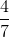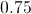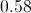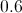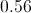Explanation:

When converting a fraction to a decimal, divide the numerator by the denominator.

Divide 4 by 7: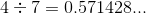Then, round to two places:

0.57

Example Question #45 : How To Find The Decimal Equivalent Of A Fraction

What isas a decimal?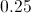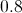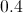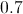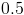Explanation:

Divide the numerator by the denominator to convert to a decimal.

2 divided by 5 is 0.4.

Example Question #46 : How To Find The Decimal Equivalent Of A Fraction

Convert the fraction to a decimal: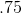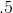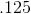Explanation:

When converting a fraction to a decimal, divide the numerator by the denominator:

Divide 1 by 2. Therefore, the decimal equivalent is 0.5.

Example Question #47 : How To Find The Decimal Equivalent Of A Fraction

Convert the fraction to a decimal. Round to two decimal places.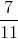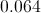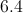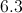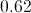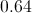Explanation:

When converting a fraction to a decimal, divide the denominator by the numerator: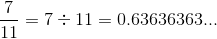Round to two decimal places. Since the thousandths place is a 6, we will round up: 0.64.

Example Question #48 : How To Find The Decimal Equivalent Of A Fraction

What isequal to in decimal form?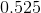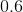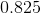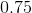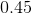Explanation:

To find the decimal equivalent of a fraction, simply divide the top number (numerator) by the bottom number (denominator). In this problem,divided byequals.

Another way to solve the problem is to try and get a multiple of ten in the denominator.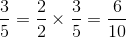Six-over-ten, or six tenths, is written as.

Example Question #49 : How To Find The Decimal Equivalent Of A Fraction

What isequal to in decimal form?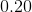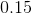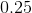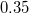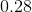Explanation:

To find the decimal equivalent of a fraction, simply divide the top number (numerator) by the bottom number (denominator). In this problem,divided byequals.

Another way to solve the problem is to try and get a multiple of ten in the denominator.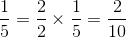Two-over-ten, or two tenths, is written as.

What is the decimal equivalent of?

.20

.08

.40

.50

.80